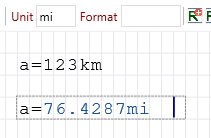# Units of measurement

Description of the calulation with units of measurement

## Units of measurement

A feature of RedCrab is the calculation with units of measure. Units can be assigned numbers, e.g. 12km or 3m2. They are predefined in RedCrab in groups such as length or area.

Mathematical expressions containing units of the same group and dimension can be added and subtracted

Multiplication and division are possible without restriction if the calculations make sense.

Examples

Make no sense

ha ∙ ha
3km / 2km

hectare * hectare
kilometre / kilometre

Correct is 3km / 2 = 1.5 km
3km + 2km = 5km kilometre + kilometre
3km + 245m = 3245m kilometre + metre
12m + 5yd = 18.123yd metre + yard
5yd + 12m = 16.572m metre + yard
4m ∙ 5m = 20m2 metre ∙ metre
2ha + 950m2 = 20950m2 hectare + square metre
650km / 5.5h = 118.18km/h Kilometre / hour

In results, the unit of the right operand is displayed. You can enter a preferred unit of measurement that will be displayed instead. For more information, see Unit of Measure Preferences.

The preferred unit of measurement is ignored if the result is incompatible.

Example
In the following example, the presetting for units is miles, the length is displayed in miles.You can enable or disable the Unit of measurement feature. The feature is disabled by default

## Define your own unit of measurement

New units can be derived from the predefined units of measure

Example

dm = 0.1m
3dm + 25cm = 55cm

## Overload the name of a unit of measurement

The names of the units can be overloaded by assigning a value to them.

For example, if m is assigned a value of 15, m is considered a variable. In this case, m can no longer be used as a unit of measurement.